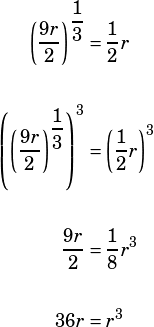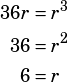If r>0 and (9r/2)^(1/3) = (1/2) r, what is the value of r?

Two good ways to go here. First, the algebra:One possible solution to the above is r = 0, but the question says that r > 0, so we can ignore that and move on:We need not consider r = –6 because we know r is positive.

The other way to go is to graph both sides of the equation and find the intersection(s).Because r is positive (my software made me use x in the graph above, as your calculator probably will, so just remember that xr), the only intersection that matters is (6, 3). At that intersection, x = 6, so we know that the value of r is 6.